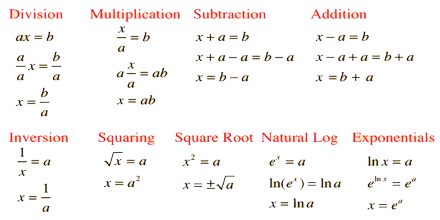Mathematic

# Algebra EquationEquation is a mathematical sentence stating that 2 expressions are equal. Example: 12 – 3 = 9; 8 + 4 = 12 etc. Prime purpose of this lecture is to present on Algebra Equation. An Equation is like a balance scale. Everything must be equal on both sides. Equals Sign (=) Means that the amount is the same on both sides. When the amounts are equal on both sides it is a true equation. When the amounts are unequal on both sides it is a false equation. When an amount is unknown on one side of the equation it is an open equation.